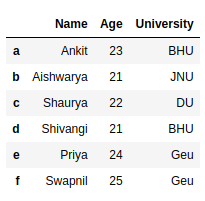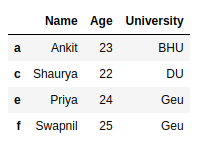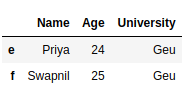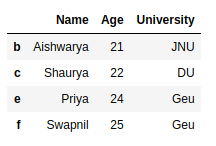# How to Drop rows in DataFrame by conditions on column values?

• Last Updated : 02 Jul, 2020

In this article, we are going to see several examples of how to drop rows from the dataframe based on certain conditions applied on a column.

Pandas provide data analysts a way to delete and filter data frame using `dataframe.drop()` method. We can use this method to drop such rows that do not satisfy the given conditions.

Let’s create a Pandas dataframe.

 `# import pandas library``import` `pandas as pd`` ` `# dictionary with list object in values``details ``=` `{``    ``'Name'` `: [``'Ankit'``, ``'Aishwarya'``, ``'Shaurya'``,``              ``'Shivangi'``, ``'Priya'``, ``'Swapnil'``],``    ``'Age'` `: [``23``, ``21``, ``22``, ``21``, ``24``, ``25``],``    ``'University'` `: [``'BHU'``, ``'JNU'``, ``'DU'``, ``'BHU'``, ``                    ``'Geu'``, ``'Geu'``],``}`` ` `# creating a Dataframe object ``df ``=` `pd.DataFrame(details, columns ``=` `[``'Name'``, ``'Age'``,``                                      ``'University'``],``                  ``index ``=` `[``'a'``, ``'b'``, ``'c'``, ``'d'``, ``'e'``,``                           ``'f'``])`` ` `df`

Output:Example 1 : Delete rows based on condition on a column.

 `# import pandas library``import` `pandas as pd`` ` `# dictionary with list object in values``details ``=` `{``    ``'Name'` `: [``'Ankit'``, ``'Aishwarya'``, ``'Shaurya'``,``              ``'Shivangi'``, ``'Priya'``, ``'Swapnil'``],``    ``'Age'` `: [``23``, ``21``, ``22``, ``21``, ``24``, ``25``],``    ``'University'` `: [``'BHU'``, ``'JNU'``, ``'DU'``, ``'BHU'``, ``                    ``'Geu'``, ``'Geu'``],``}`` ` `# creating a Dataframe object ``df ``=` `pd.DataFrame(details, columns ``=` `[``'Name'``, ``'Age'``,``                                      ``'University'``],``                  ``index ``=` `[``'a'``, ``'b'``, ``'c'``, ``'d'``, ``'e'``, ``'f'``])`` ` `# get names of indexes for which``# column Age has value 21``index_names ``=` `df[ df[``'Age'``] ``=``=` `21` `].index`` ` `# drop these row indexes``# from dataFrame``df.drop(index_names, inplace ``=` `True``)`` ` `df`

Output :Example 2 : Delete rows based on multiple conditions on a column.

 `# import pandas library``import` `pandas as pd`` ` `# dictionary with list object in values``details ``=` `{``    ``'Name'` `: [``'Ankit'``, ``'Aishwarya'``, ``'Shaurya'``, ``              ``'Shivangi'``, ``'Priya'``, ``'Swapnil'``],``    ``'Age'` `: [``23``, ``21``, ``22``, ``21``, ``24``, ``25``],``    ``'University'` `: [``'BHU'``, ``'JNU'``, ``'DU'``, ``'BHU'``,``                    ``'Geu'``, ``'Geu'``],``}`` ` `# creating a Dataframe object ``df ``=` `pd.DataFrame(details, columns ``=` `[``'Name'``, ``'Age'``,``                                      ``'University'``],``                  ``index ``=` `[``'a'``, ``'b'``, ``'c'``, ``'d'``, ``'e'``, ``'f'``])`` ` `# get names of indexes for which column Age has value >= 21``# and <= 23``index_names ``=` `df[ (df[``'Age'``] >``=` `21``) & (df[``'Age'``] <``=` `23``)].index`` ` `# drop these given row``# indexes from dataFrame``df.drop(index_names, inplace ``=` `True``)`` ` `df`

Output :Example 3 : Delete rows based on multiple conditions on different columns.

 `# import pandas library``import` `pandas as pd`` ` `# dictionary with list object in values``details ``=` `{``    ``'Name'` `: [``'Ankit'``, ``'Aishwarya'``, ``'Shaurya'``,``              ``'Shivangi'``, ``'Priya'``, ``'Swapnil'``],``    ``'Age'` `: [``23``, ``21``, ``22``, ``21``, ``24``, ``25``],``    ``'University'` `: [``'BHU'``, ``'JNU'``, ``'DU'``, ``'BHU'``, ``                    ``'Geu'``, ``'Geu'``],``}`` ` `# creating a Dataframe object ``df ``=` `pd.DataFrame(details, columns ``=` `[``'Name'``, ``'Age'``,``                                      ``'University'``],``                  ``index ``=` `[``'a'``, ``'b'``, ``'c'``, ``'d'``, ``'e'``, ``'f'``])`` ` `# get names of indexes for which``# column Age has value >= 21``# and column University is BHU``index_names ``=` `df[ (df[``'Age'``] >``=` `21``) & (df[``'University'``] ``=``=` `'BHU'``)].index`` ` `# drop these given row``# indexes from dataFrame``df.drop(index_names, inplace ``=` `True``)`` ` `df`

Output :My Personal Notes arrow_drop_up